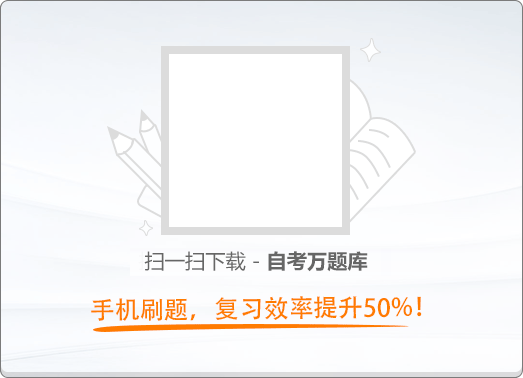150:002018年4月《大学语文》真题

1
(单项选择题)

• A.

蓝田日暖玉生烟

• B.

僧推月下门

• C.

烟光凝而暮山紫

• D.

惟陈言之务去

• A
• B
• C
• D

2
(单项选择题)

《就任北京大学校长之演说》：“平时则放荡冶游，考试则熟读讲义，不问学问之有无，惟争分数之多寡。”这里采用的修辞手法是（　）。

• A.

排比

• B.

层递

• C.

对偶

• D.

借代

• A
• B
• C
• D

3
(单项选择题)

《如何避免愚蠢的见识》一文中，罗素认为，对于富于心理想象力的人来说，避免愚蠢的见识的“一个好办法便是设想一下自己在与一位怀有不同偏见的人进行辩论”，对此展开论说时，他所提到的见识是（　）。

• A.

亚里士多德误以为妇女牙齿的数目比男人少

• B.

某个人硬要说二加二等于五，或者说冰岛位于赤道

• C.

圣雄甘地就对铁路、轮船和机器深表遗憾

• D.

不论男人或女人，十之八九都坚信男性或女性特别优越

• A
• B
• C
• D

4
(单项选择题)

• A.

天之亡我，我何渡为

• B.

反我何面目见之

• C.

时不利兮骓不逝

• D.

是何楚人之多也

• A
• B
• C
• D

5
(单项选择题)

• A.

嫉恶如仇

• B.

从容镇定

• C.

宽厚谦和

• D.

博闻强记

• A
• B
• C
• D

6
(单项选择题)

• A.

能顺木之天，以致其性焉尔

• B.

其莳也若子

• C.

则又爱之太殷，忧之太勤

• D.

其置也若弃

• A
• B
• C
• D

7
(单项选择题)

• A.

《寡人之于国也》

• B.

《五代史伶官传序》

• C.

《张中丞传后叙》

• D.

《赵武灵王胡服骑射》

• A
• B
• C
• D

8
(单项选择题)

• A.

寄蜉蝣于天地

• B.

渺沧海之一粟

• C.

挟飞仙以遨游

• D.

抱明月而长终

• A
• B
• C
• D

9
(单项选择题)

• A.

轻丝

• B.

弱管

• C.

蛾冠

• D.

竹肉

• A
• B
• C
• D

10
(单项选择题)

• A.

后来知道他的别号就叫“怒庵”，也就不以为奇

• B.

这种家庭教育是相当危险的，没有几个人能成功，然而傅雷成功了

• C.

他才成为具有浩然之气的儒家之刚者

• D.

朋友中像傅雷那样的毅然决然不自惜其生命的，还有好几个

• A
• B
• C
• D

11
(单项选择题)

• A.

诸葛亮

• B.

李冰

• C.

刘备

• D.

杜甫

• A
• B
• C
• D

12
(单项选择题)

《我与地坛》反复抒写“我”对母亲的思念、痛悔之情，从而展现母爱的动人力量，这种表现方式是（　）。

• A.

侧面烘托

• B.

铺陈排比

• C.

类比

• D.

递进

• A
• B
• C
• D

13
(单项选择题)

• A.

桂栋兮兰橑，辛夷楣兮药房

• B.

捐余袂兮江中，遗余褋兮澧浦

• C.

白玉兮为镇，疏石兰兮为芳

• D.

合百草兮实庭，建芳馨兮庑门

• A
• B
• C
• D

14
(单项选择题)

• A.

被征召入长安之前

• B.

被征召入长安之时

• C.

被迫离开长安之后

• D.

被判流放夜郎之时

• A
• B
• C
• D

15
(单项选择题)

• A.

金河秋半虏弦开，云外惊飞四散哀

• B.

仙掌月明孤影过，长门灯暗数声来

• C.

须知胡骑纷纷在，岂逐春风一一回

• D.

莫厌潇湘少人处，水多菰米岸莓苔

• A
• B
• C
• D

16
(单项选择题)

《鹧鸪天》（重过阊门万事非）中借典故喻丧偶之痛的句子是（　）。

• A.

梧桐半死清霜后

• B.

头白鸳鸯失伴飞

• C.

已旧栖新垅两依依

• D.

空床卧听南窗雨

• A
• B
• C
• D

17
(单项选择题)

《再别康桥》中，“康桥”所在的国度是（　）。

• A.

中国

• B.

英国

• C.

法国

• D.

美国

• A
• B
• C
• D

18
(单项选择题)

• A.

河边上破旧的老水车

• B.

淤滩上的驳船

• C.

未落到地面的花朵

• D.

雪被下古莲的胚芽

• A
• B
• C
• D

19
(单项选择题)

• A.

心里因想道：“别人不知道我的心，还可恕；连他也奚落起我来。”

• B.

心里想着：“你心里自然有我，虽有‘金玉相对’之说，你岂是重这邪说不重人的呢？”

• C.

心中又想着：“我不管怎么样都好，只要你随意，我就立刻因你死了，也是情愿的。”

• D.

又自己后悔：“方才不该和他较证，这会子他这样光景，我又替不了他。”

• A
• B
• C
• D

20
(单项选择题)

《哦，香雪》：“可在这儿，和同桌的铅笔盒一比，为什么显得那样笨拙、陈旧？它在一阵哒哒声中有几分羞涩地畏缩在桌角上。”这里的“它”指的是（　）。

• A.

有机玻璃发卡

• B.

柳条篮子

• C.

人造革学生书包

• D.

小木盒

• A
• B
• C
• D